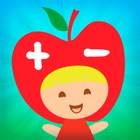• 검색 유형

모든 iPhone 애플리케이션 카테고리

모든 iPhone 게임 카테고리# Math Minis

15 8

8 사용자
평가 등급

## 스크린샷

설명

Math Minis – Addition and Subtraction.

More fun than a math workbook - select the correct answer and the fruit comes alive with cute animation. Features 8 different levels of adding and subtracting skill sets. Each level has a different background, tree and fruits that turn into little people. Perfect for preparing preschooler into their first year of elementary school.

Level 1 – Counting and Number Patterns
• Counting Review
• Number Sequence
• Number Patterns

• Add 3 single digit numbers

• Add 2 digits to 1 digit numbers
• Add 2 digits to 2 digit numbers
• Fill in the missing number

Level 4 – Basic Subtraction
• Subtract single digit numbers
• Subtract 3 single digit numbers
• Subtracting 10s

• Subtract 1 digit from 2 digit numbers
• Subtract 2 digits from 2 digit numbers
• Fill in the missing number

Level 6 – Addition and Subtraction Review
• Mixed questions of addition and subtraction with single digit numbers
• Mixed questions of addition and subtraction with 3 single digit numbers
• Mixed questions of adding and subtracting 10s

Level 7 – Combining Addition and Subtraction
• Add and subtract single digit number
• Add and subtract 1 and 2 digit numbers
• Fill in the missing number

Level 8 – Final Challenge
• Add 2 digits to 2 digit numbers
• Subtract 2 digits from 3 digit numbers
• Fill in the missing number

The first 4 levels are free.
If you like this app, visit http://www.ripplepublishing.ca for more fun apps

: 15 리뷰

"우수"

8